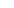JOIN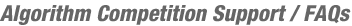Algorithm Competition Rating System
Algorithm Competition Rating SystemRating Key2200+ 1500 - 2199 1200 - 1499 900 - 1199 0 - 899A coder's handle is color-coded depending on their rating within the Competition Arena.How the TopCoder Rating System Works: The following statistics are kept about each coder: Rating Volatility Number of times previously rated Before competing, new members ratings are provisional. After a competition the algorithm below is applied to the competitors. First, the ratings of members who have previously competed are calculated, with new members' performances not considered. Second, new members are given a rating based on their performance relative to everyone in the competition.How algorithm ratings are calculated

New ratings are calculated as follows:

After each competition, each coder who attended the competition is re-rated according to the following algorithm. Keep in mind that only coders who shared the same problem set are rated against one another. The average rating of everyone in the competition is calculated:Where NumCoders is the number of coders in the competition and Rating is the rating without the volatility of the coder in the competition before the competition.

The competition factor is calculated:Where Volatility is the volatility of the coder in the competition before the competition.

Win Probability Estimation Algorithm: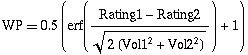Where Rating1 & Vol1 are the rating and volatility of the coder being compared to, and Rating2 & Vol2 are the rating and volatility of the coder whose win probability is being calculated. Erf is the "error function".

The probability of the coder getting a higher score than another coder in the competition (WPi for i from 1 to NumCoders) is estimated. The expected rank of the coder is calculated: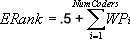The expected performance of the coder is calculated:Whereis the inverse of the standard normal function.

The actual performance of each coder is calculated: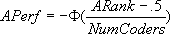Where ARank is the actual rank of the coder in the competition based on score (1 for first place, NumCoders forlast). If the coder tied with another coder, the rank is the average of the positions covered by the tied coders.

The performed as rating of the coder is calculated:The weight of the competition for the coder is calculated:Where TimesPlayed is the number of times the coder has been rated before.

To stabilize the higher rated members, the Weight of members whose rating is between 2000 and 2500 is decreased 10% and the Weight of members whose rating is over 2500 is decreased 20%.

A cap is calculated: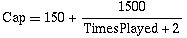The new volatility of the coder is calculated:The new rating of the coder is calculated:If |NewRating - Rating| > Cap the NewRating is adjusted so it is at most Cap different than Rating.